# AP Statistics : How to use boxplots to summarize a data set

## Example Questions

### Example Question #31 : Data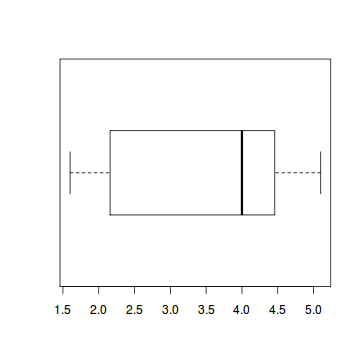Which of the following is true based on the box plot?

i. The mean is.

ii. The range is approximately.

iii. The IQR is approximatly.

ii and iii

i,ii, and iii

i and ii

ii only

iii only

iii only

Explanation:

The median, not the mean is. The IQR is about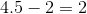. The range is about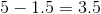.

### Example Question #1 : How To Use Boxplots To Summarize A Data Set

Median

Mean

Mode

Interquartile range

Range

Median

Explanation:

A box and whisker plot separates the data into quartiles so that each quartile has an equal number of data points. The box indicates the interquartile range, that is, the top line of the box is the third quartile and the bottom line of the box is the second quartile. The line separating the second and third quartiles indicates the median. The lines outside of the box indicate the outer-quartiles (first and fourth).

### Example Question #33 : Data

A distribution has a minimum of, a first quartile of, a median of, a third quartile ofand a maximum of. Which of the following are true?

1. The interquartile range is.
2. The distribution is skewed left.
3. The range is.

1 only

2 and 3

1, 2 and 3

1 and 3

1 and 2

1 and 3

Explanation:

1) and 3) come easily because of straightforward calculations - it's 2 that's the tough part.

1) is true because the interquartile range is defined as the third quartile minus the first quartile.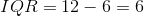3) is true because the range of a data set is the difference of the maximum value and the minimum value.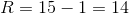Notice that the median is closer to the first quartile, indicating that the distribution is skewed right. (If the median were closer to the third quartile, that would indicate a distribution that is skewed left.) It can help to draw the boxplot of the data set in order to visualize it.

### Example Question #34 : Data

Which data set could be represented by the following boxplot?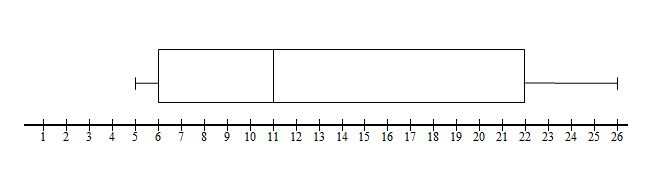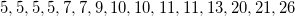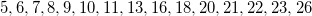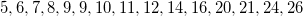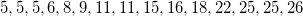Explanation:

This boxplot is representing a data set with a median of 11. The data sethas a median of 13, so we can eliminate this choice. And while the data setdoes have a mean of 11, its median is 10.

To determine which of the remaining choices matches the boxplot, find the first and third quartiles. To find the first quartile, find the median of the lower half, excluding the median, in each case 11.has a first quartile of 8has a first quartile of 6

The boxplot shown has the lower end of the rectangle at 6, so the last data set listed is the correct answer.

### Example Question #1 : How To Use Boxplots To Summarize A Data Set

Which data set would be represented by this boxplot?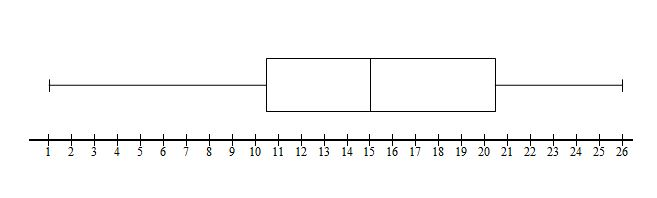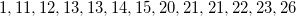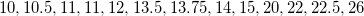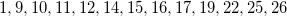Explanation:

A boxplot indicates the quartiles of the data set. In this case we can see that the median or second quartile is 15. We can immediately eliminateas a choice, since its median is 13.75, not to mention its smallest value is 10 rather than 1. The other choices all go from 1 to 26 like the boxplot indicates.

Now we need to find the data set with the correct first and third quartile. The boxplot indicates that our first quartile is 10.5. We can find Q1 by finding the median of the lower half, not including the median:has Q1 of 12.5, found by taking the mean of 12 and 13. We can eliminate this choice.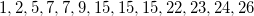has a Q1 of 6, found by taking the mean of 5 and 7. We can eliminate this choice.has a Q1 of 10.5, which is exactly what we want. We can also verify that the median of the top half is 20.5.

### Example Question #2 : How To Use Boxplots To Summarize A Data Set

Which statement about the data represented by this boxplot is not true?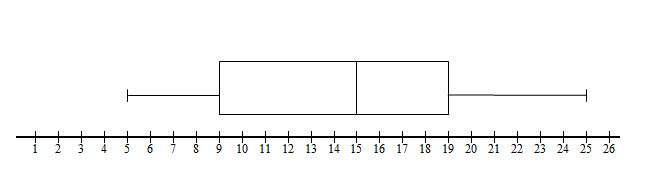The range is exactly twice the interquartile range.

The interquartile range is 10.

The median is 15.

The third quartile is 9.

The third quartile is 9.

Explanation:

In order to get this question right, we need to be able to distinguish between the first and the third quartile.

The third quartile, 19, is the median of the upper half of the data.

The first quartile, 9, is the median of the lower half of the data.

This means that the "correct" wrong statement is "the third quartile is 9," since 9 is the first quartile.